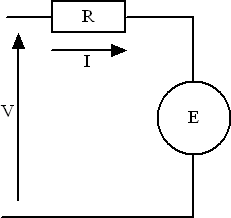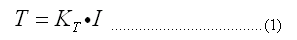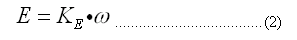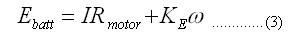A brushed DC motor of the type likely to be used in a micromouse is modeled as a combination of an ideal motor with a built in series resistance, Rmotor.

The ideal motor turns at a speed determined by the voltage (emf) applied to it and provides a torque proportional to the current passing through it. Since the motor windings have a finite resistance, some energy is lost in the windings. There is also a limit to the current that can pass through the motor for a given applied voltage and hence the amount of torque that it can develop.Motors have an armature that will have a particular moment of inertia. even with no outside load, a motor will take a finite amount of time to run up to speed because of that inertia and because of friction present in the bearings. For most purposes the frictional losses should be small enough to ignore.

Motor Equations

If you hold the shaft of a DC motor stationary and apply a voltage to the terminals a torque will be developed in the motor. The value of that torque is the stall torque for the motor and is dependent only upon the current flowing and the characteristics of the particular motor. Another way to examine the characteristic of a motor is to spin the armature at a known speed and measure the open circuit emf generated at the terminals.

These two tests allow us to write an important pair of equations that describe the behaviour of a DC motor:Torque, T, is measured in Nm
Current, I, is in Amps
EMF, E, is in Volts and
Angular velocity, w, is in radians/sec

The units for KT are Nm/Amp and for KV, they are Volts per radian per second

If you are not happy with radians per second then multiply RPM by 2*pi/60 to get rad/sec. If you ensure that you use the correct units you will find that KE and KT have the same value. Thus, if you can measure one, you will know the value of the other.

Running at Constant Speed

If you connect a real motor to a battery, you will find that it will spin up to a speed that depends upon the battery voltage. It cannot just go faster and faster because the spinning of the armature generates a voltage, the back emf, that opposes the applied voltage. For the motor to spin at all, there must be some current flowing. That current must also flow in the motor resistance and so there will be a small voltage drop (IxR) across that internal resistance. Now we have an equation for the actual speed of the motor when a voltage is applied to it:Consider an actual motor – the RF300 type common in cassette and CDROM players. At its rated voltage of 3V, the stall torque is given as 0.0024 Nm at a current of 0.265 Amps. Thus the value of KT is 0.0024/0.265 = 0.0091 Nm/Amp. The motor resistance is R = V/I = 3/0.265 = 11.3 Ohms.

Now we connect the motor to a 3 Volt supply that has a negligible internal resistance. The motor spins up, drawing just enough current to proved sufficient torque to overcome internal losses. That current is measured at 0.022 Amps. Solving the motor equation (3) we get

3 = 0.022 *11.3 + 0.0091*w
w = (3-0.022*11.3)/0.0091
= 2900 RPM

We can calculate the motor torque under these conditions from the equation above. In this case it will be T = KTI = 0.0091*0.022 = 0.2mNm.

Suppose we load up the motor with some additional load, keeping the appled voltage the same. As the torque increases, the armature slows down, the back emf decreases and so the current rises. At some point the current rises sufficiently to generate a torque that exactly matches the load and the speed stabilises again. Now, however, there will be a higher current flowing through the internal resistance and power will be wasted just heating up the motor. In the ultimate case, the motor will be stalled and all the current will be heating the windings. For our example motor, at 3V the motor dissipates 0.8 Watts as heat. Not a great deal but the heating effect is one of the factors that will limit the top speed of the motor. Other factors include the frictional and dynamic losses in the motor (and any attached drive system), the quality of the bearings, the anticipated motor life as well as losses in the commutator. Should we wish to run this motor at 12 Volts, its stall current would become 1.06 Amps and the motor would dissipate nearly 13 Watts – as much as a night light. It would probably not last long under those circumstances

Still, free running at 12 volts (if the bearings are good for it) would, in principle, have the motor running at a fair speed. More torque is needed to overcome the mechanical losses and this particular motor draws 0.085 Amps at 12 Volts. Repeating the previous calculation indicates it should be running at about 11,600 RPM. I don’t suppose it will last long but they are cheap and the average micromouse does not expect to run for hours.

Acceleration

There is a separate page about selecting an appropriate gear ratio for your mouse. Assume for now we have chosen a gear ratio and wheel size for the mouse. For the purpose of illustration we will use the following values:

Gear Ratio, G = 5
Gear efficiency, Eff = 0.8
Wheel Diameter, D = 0.035 m
Mass of mouse, M = 0.4 kg
Number of motors = 2
Intended acceleration, A = 4m/s/s

The torque at the wheels, TW, required for this acceleration is readily calculated from Newtons equations

F = MA and TW = F * D/2
F = 0.4*4 = 1.6 N
TW = 1.6 * 0.035/2 = 0.028 Nm

The gearing means that the motors only need to provide a fraction of that, plus a bit to overcome efficiency losses in the gear train. There are two motors and they share the load equally. Thus the required torque for each motor, TM, is

TM = TW/G/Eff/2
TM = 0.028 / 5 / 0.8 / 2= 0.0035 Nm

From the earlier motor equations, we can calculate the current required for each motor, IM, to provide that torque:

IM = TM/KT
IM = 0.0035 / 0.0091 = 0.385 Amps

Simply applying 4.5 Volts across the motor will produce that torque at startup. However, as the speed increases, the back emf also increases, the motor current drop and acceleration is reduced. We really want to be able to produce those kinds of accelerations even while travelling at speed.

A fairly typical NiMH, AAA cell of 750 mAhr might have an internal resistance of about 0.1 Ohm. A battery consists of a number of cells in series. A battery made from 10 of these cells would have a total internal resistance, Rbatt, of 1 Ohm. Current flowing through the motor also flows through this resistance and so, the higher the current we want, the greater will be the loss due to the internal resistance of the battery so reducing the available voltage to drive the motor. Suppose for now that we have no additional losses in the motor driver, although a typical bipolar driver may cost us another Volt or two

So, we want to know how fast we can go while still accelerating at our nominal 4m/s/s. In order to produce the torque required, our motor needs 0.385 Amps. That current must flow through the motor resistance so we must have

(11.3 * 0.385) = 4.34 Volts

across the motor.

Both motors will be running so the loss from the battery will be

2 * Rbatt * Im Volts
= 2 * 1 * 0.385
= 0.77 Volts.

Now, the remaining voltage available from the battery can all be taken up by the back emf of the rotating motor. In this case it will be

(10 * 1.2 – 4.34 – 0.77) = 6.89 Volts

From the voltage constant equation for the motor we can calculate how fast the motor will be turning when the back emf is 6.89 Volts:

w = E/KE = 6.89/0.0091 = 757 rad/sec = 120 revolutions per second

This means the wheels will be turning at 120/5 = 24 revolutions per second and with a wheel diameter of 0.035 m, our mouse should be doing

3.14 * 0.035 * 24 = 2.65 m/s

Which is pretty good

Top Speed

The final top speed of the mouse will be somewhat higher than this value and is a little more difficult to be confident of. Again, as the speed increases, the available current reduces until the system is in equilibrium with only just enough current being drawn to overcome losses in the mouse. Such losses are difficult to calculate and I would suggest that the only way to know would be to have the mouse monitor actual current and keep a record. This running current should be much less than the current required during acceleration but will be more than the unloaded current measured above. Suppose it is 0.120 mA for this particular configuration.

Each motor would then drop

0.120 * 11.3 = 1.35 Volts

as a result of its internal resistance.

Battery losses will be

2 * 0.12 * 1 = 0.24 Volts

leaving us with

12 – 1.35 – 0.24 = 10.41 Volts

for the motor back emf. This corresponds to a speed of 1144 rad/sec at the motor and (1144/5/6.28)*(3.14*0.035) = 4 m/s for the mouse.

I have made a spreadsheet in Excel with these calculations (and a few more) so that you can try out figures for your motors and mouse.